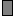#State

## Interpretation

A state is a model value that is changed by events, but otherwise stays the same. A state does not have a unique equation, but rather a set of rules, each consisting of an event (the trigger) matched with an equation (the effect). There will be one rule for each event that influences the state, plus an extra effect labelled "reset..." that sets the initial value of the state when the model resets (or when a new instance of the state's parent submodel is created).

In the equation dialogue for a state, the influencing event symbol captions (and reset...) are listed to the left of the equation field, and clicking on an event caption shows the effect for an occurrence of that event. In the equation bar, a pull-down menu to the left selects the caption of the event whose effect is to be edited. The popup text for the state is a condensed form of the rules, e.g., "9 on event1, 6 on event2, 0 on reset."

Components with continuous values can also influence the state, and their values can be used in one or more of its effect equations.

The value of a state after an event occurrence depends only on the effect equation of that event, and not on its previous value. However the equation can use the prev(0) function to refer to the state's previous value if the new value is to be a function of it. The equation can also use the trigger_magnitude() function to refer to the magnitude of the triggering event occurrence if this is to affect the state's new value. The effect equations cannot use the after(t,x) function; the state's value always changes immediately after the triggering event occurs.

Currently the order in which the rules are applied if multiple triggerring events occur simultaneously is undefined.

## Rules

• A state must have influences from at least one event or squirt symbol
• A state must have an equation for each event or squirt symbol influencing it, plus one for reset
• Other components (including other states) can influence it, and their values can be used in its equations
• All of its equations must evaluate to quantities with the same units and dimensions
• A state can influence any other component